Home > CCA2 > Chapter 9 > Lesson 9.3.3 > Problem9-106

9-106.
1. Use the properties of logarithms to rewrite each expression as an equivalent one using only one logarithm. Homework Help ✎

1. log3(5) + log3(m)

2. log6(p) − log6(m)

3. log2(r) + 3log6(z)

4. log(90) + log(4) − log(36)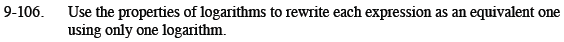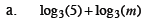Review the Math Notes box in Lesson 6.2.2.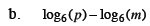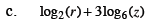Careful! Look at the bases.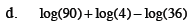log(10) = ?